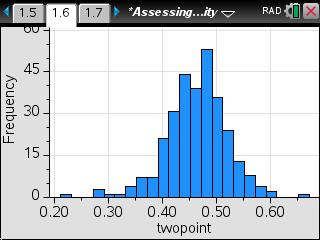### Assessing Normality

In this activity, students will learn four characteristics of a normal curve: the distribution is symmetric and mound-shaped; the mean and median are approximately equal; the distribution meets the 68-95.5-99.7 rule; and the normal probability plot is linear. They will use these to determine if a data set it normal.
•TI-Nspire™ CX/CX II
•TI-Nspire™ CX CAS/CX II CAS
• TI-Nspire™ Navigator™
• TI-Nspire™
• TI-Nspire™ CAS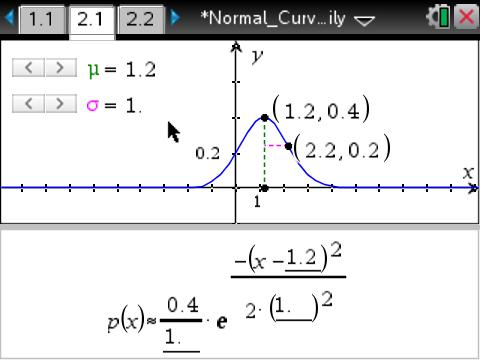### Exploring the Normal Curve Family

Students will investigate the relationship of the equation of a normal curve to its graph. They will use a slider to change the values of two parameters, μ and σ, to investigate their effects on the normal curve, noting in particular that μ represents the location of the mean and that σ represents the distance from the mean to the curve at the point of inflection.
•TI-Nspire™ CX/CX II
•TI-Nspire™ CX CAS/CX II CAS
• TI-Nspire™ Navigator™
• TI-Nspire™
• TI-Nspire™ CAS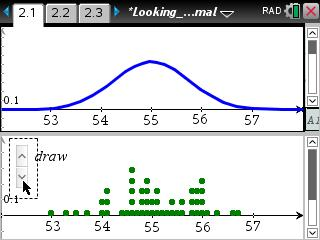### Looking Normal

This lesson involves examining multiple samples taken from a single approximately normal population.
•TI-Nspire™ CX/CX II
•TI-Nspire™ CX CAS/CX II CAS
• TI-Nspire™ Navigator™
• TI-Nspire™
• TI-Nspire™ CAS### Normal Probability Plot

This lesson involves creating a normal probability plot for several data sets involving height to examine the appearance of such plots when the distribution is approximately normal.
•TI-Nspire™ CX/CX II
•TI-Nspire™ CX CAS/CX II CAS
• TI-Nspire™ Navigator™
• TI-Nspire™
• TI-Nspire™ CAS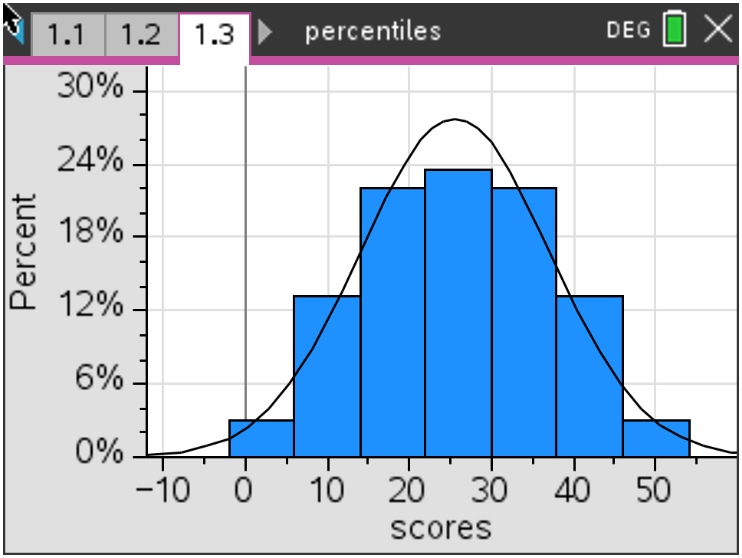### Percentiles

The goal of this activity is for students to use the area to the left of a value in a normal distribution to find its percentile. The process will then be reversed to find the value for a given percentile.

•TI-Nspire™ CX/CX II
•TI-Nspire™ CX CAS/CX II CAS
• TI-Nspire™ Navigator™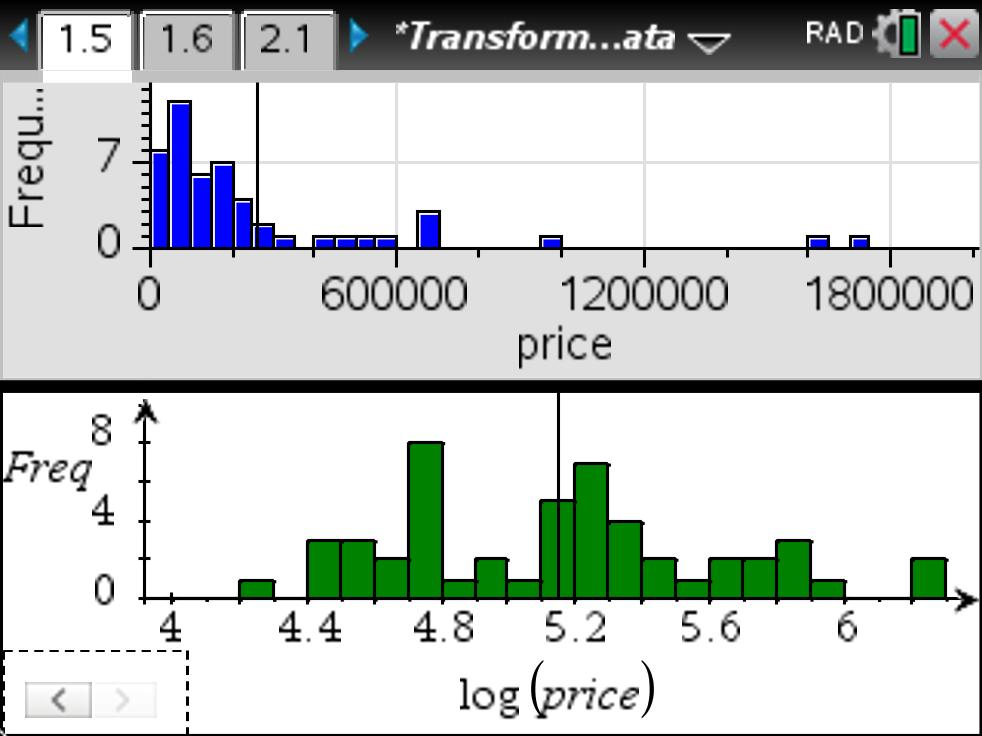### Transforming Univariate Data

This lesson involves square root, logarithmic, square, and exponentiation transformations of skewed univariate data using a given data set.
•TI-Nspire™ CX/CX II
•TI-Nspire™ CX CAS/CX II CAS
• TI-Nspire™ Navigator™
• TI-Nspire™
• TI-Nspire™ CAS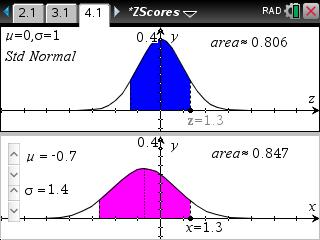### Z-Scores

This lesson involves finding the area under the standard normal curve with mean 0 and standard deviation 1 for a given distance from the mean and compare this to the area under the curve for another member of the family of normal curves.
•TI-Nspire™ CX/CX II
•TI-Nspire™ CX CAS/CX II CAS